# What Is a Force? Mass, Velocity, Acceleration and Adding Vectors

Eugene is a qualified control/instrumentation engineer Bsc (Eng) and has worked as a developer of electronics & software for SCADA systems.

In this first part of a two part tutorial, we'll learn the absolute basics about forces!

What's covered:

• Definitions of force, mass, velocity, acceleration, weight
• Vector diagrams
• Adding and resolving vectors

## What is a Force?

We can think of a force of a force as a push or pull.

## What Are Examples of Forces?

• When you lift something off the ground, your arm is exerting a force upwards on the object. This is an example of an active force
• The Earth's gravity pulls down on an object and this force is called weight
• A bulldozer can exert a huge force, pushing material along the ground
• A huge force or thrust is produced by the engines of a rocket lifting it up into orbit
• When you push against a wall, the wall pushes back. If you try to compress a spring, the spring tries to expand and pushes back against your hand. When you stand on the ground, it pushes up and supports you. All these are examples of reactive forces. They don't exist without an active force.
• If the unlike poles of two magnets are brought together (N and S), the magnets will attract each other. However, if two like poles are moved close together (N and N or S and S), the magnets will repel

## Fundamental Quantities Used in Physics

Before we learn any more details about forces, we need to become familiar with the international system of units, abbreviated to SI from the French Système International d’Unités. It's the units system used for engineering and scientific calculations and is basically a standardization of the metric system.

3 of the 7 base quantities in the SI system are used in the study of mechanics (a branch of physics concerned with forces and motion). They are mass, length and time.

Mass

This is a property of a body (any object) and a measure of the body's resistance to motion known as inertia. It is constant and has the same value no matter where an object is located on Earth, on another planet or in space. The unit of mass in the SI system is the kilo (kg).

Length

In the context of mechanics, the distance travelled by an object. The unit of length or distance is the metre (m)

Time

The time taken for an object to travel a given distance. The unit of time is the second (s)

Scroll to Continue

## Read More From Owlcation

### Derived Quantities

These quantities can be derived from mass, length and time. The basic derived quantities are:

Velocity

This is the speed of a body in a given direction and is measured in metres per second (m/s). Average velocity equals distance travelled (length) divided by time taken.

Acceleration

When a force is exerted on a mass, it accelerates. In other words, the velocity increases. This acceleration is greater for a greater force or for a smaller mass. Acceleration is measured in metres per second per second or metres per second squared (m/s²). Average acceleration equals change in velocity divided by the time the change occurred in.

Force

This can be thought of as a “push” or “pull.” A force can be active or reactive. Force equals mass multiplied by acceleration. The unit of force is the newton (N) (We’ll learn more about this later)

Note: In US English, metres is spelled “meters”.

## What One Newton Feels Like

Force in the SI system of units is measured in newtons (N). A force of 1 newton is equivalent to a weight of about 3.5 ounces or 100 grams. It's also defined as the force that will give a mass of 1kg an acceleration of 1 metre per second per second. We'll discover more about this in the second part of this tutorial.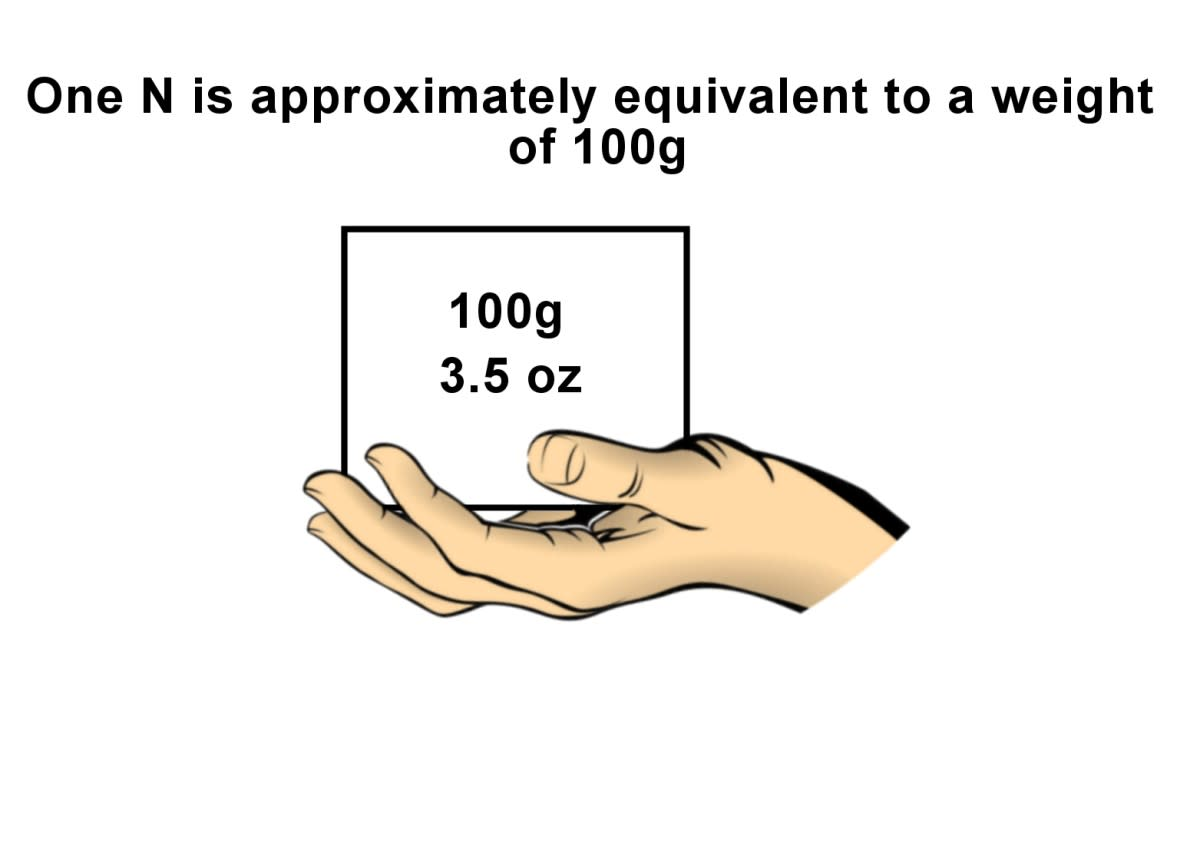One N is equivalent to about 100g or 3.5 ounces, a little more than a pack of playing cards.© Eugene Brennan

## A Very Large Force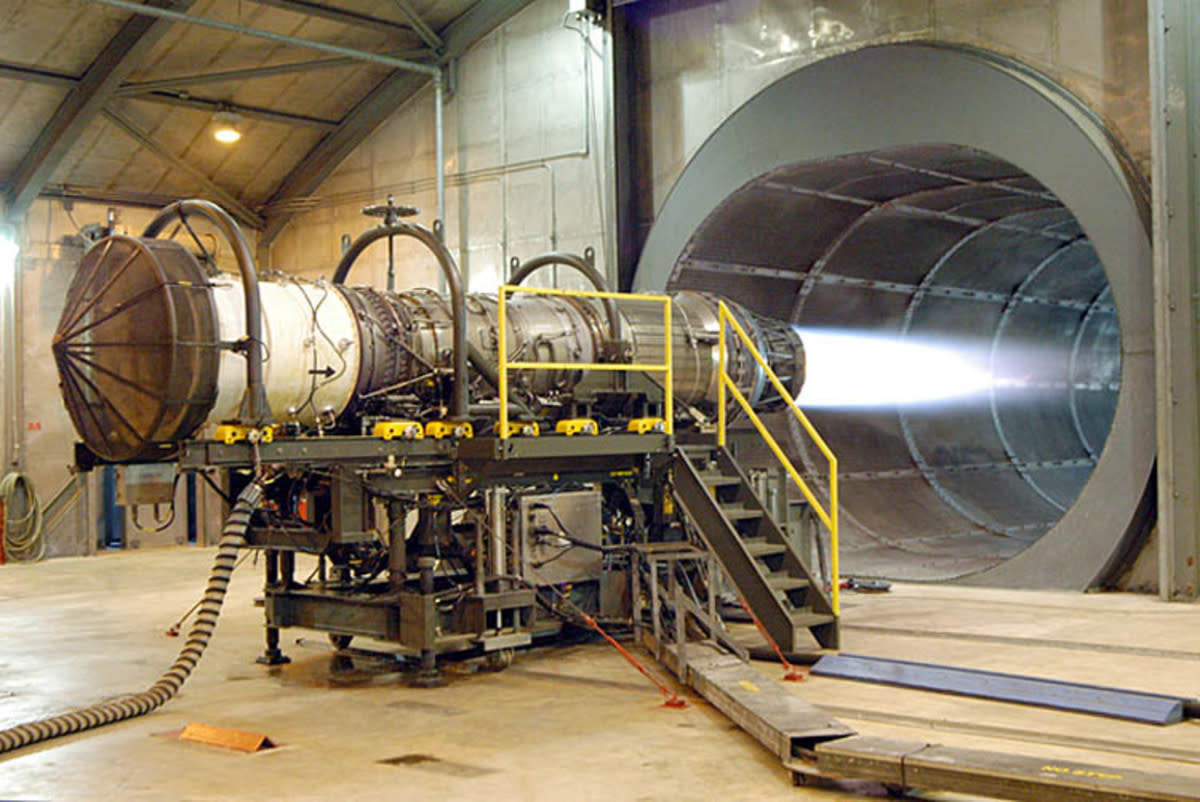A Pratt & Whitney turbofan engine as used on the F15 fighter jet. This engine develops a thrust of 130 kN (equivalent to a weight of 13 tonnes)U.S. Air Force photo by Sue Sapp, public domain via Wikimedia Commons

## What Types of Forces Are There?

### Effort, Thrust and Tractive Force

Different words are used depending on context to refer to the the force involved when a human or machine moves an object. Effort is often used to refer to the force applied to an object which may eventually cause it to move. For example, when you push or pull a lever, slide a piece of furniture, turn a nut with a wrench, or a bull dozer pushes a load of soil, the applied force is called an effort. When a vehicle is driven forwards by an engine, or carriages are pulled by a locomotive, the force which causes motion and overcomes friction and air drag is known as traction or the tractive force. For rocket and jet engines, the term thrust is often used.

### Weight

This is the force exerted by gravity on an object. It depends on the mass of the object and varies slightly depending on where it is located on the planet and the distance from the centre of the Earth. An object's weight is less on the Moon and this is why the Apollo astronauts seemed to bounce around a lot and could jump higher. However it could be greater on other planets. Weight is due to the gravitational force of attraction between two bodies. It is proportional to the mass of the bodies and inversely proportional to the square of the distance apart.

### Tensile or Compressive Reaction

When you stretch a spring or pull on a rope, the material undergoes a strain or internal distortion that results in an equal reactive force pulling back in the opposite direction. This is known as tension and is due to stress caused by displacement of molecules in the material. If you try to compress an object such as a spring, sponge or gas, the object pushes back. Again this is due to strain and stress in the material. Working out the magnitude of these forces is important in engineering so that structures can be built with members which will withstand the forces involved, i.e they won't stretch and snap, or buckle under load.

### Static Friction

Friction is a reactive force which opposes motion. Friction can have beneficial or detrimental consequences. When you try to push a piece of furniture along the floor, the force of friction pushes back and makes it difficult to slide the furniture. This is an example of a type of friction known as dry friction, static friction or stiction.
Friction can be beneficial. Without it everything would slide and we wouldn't be able to walk along a pavement without slipping. Tools or utensils with handles would slide out of our hands, nails would pull out of timber and brakes on vehicles would slip and not be of much use.

### Viscous Friction or Drag

When a parachutist moves through the air or a vehicle moves on land, friction due to air resistance, slows them down. Air friction also acts against an aircraft as it flies, requiring extra effort from the engines. If you try to move your hand through water, the water exerts a resistance and the quicker you move your hand, the greater the resistance. The same thing happens as a ship moves through water. These reactive forces are known as viscous friction or drag.

### Buoyancy Force

This is the force which pushes up on an object immersed in a fluid (a fluid can be a gas or liquid). So for instance when you push a ball down into water, you can feel the buoyancy force pushing it back upwards. You can read more about buoyancy and the Archimedes' principle in this tutorial:
Archimedes' Principle Experiments and Buoyant Force

### Electrostatic and Magnetic Forces

Electrically charged objects can attract or repel each other, similar to how poles of a magnet will repel each other while opposite poles will attract. Electric forces are used in powder coating of metal and electric motors work on the principle of magnetic forces on electric conductors.Types of forces.© Eugene Brennan. Individual public domain clipart images sourced from Publicdomainvectors.com

## What Is a Load?

When a force is exerted on a structure or other object, this is known as a load. Examples are the weight of a roof on the walls of a building, the force of wind on a roof, or the weight pulling down on the cable of a crane when hoisting.

## What Is a Vector?

A vector is a quantity with magnitude and direction. Some quantities such as mass don't have a direction and are known as scalars. However, velocity is a vector quantity because it has a magnitude called speed and also direction (i.e., the direction an object is travelling). Force is also a vector quantity. For example, a force acting down on an object is different from a force acting upwards on the underside.

Vectors are graphically represented on diagrams by an arrow, with the angle of the arrow wrt a reference line representing the angle of the vector. We can solve mechanics problems by drawing vectors to scale with the length of the arrow representing its magnitude and then adding the vectors to find unknown forces.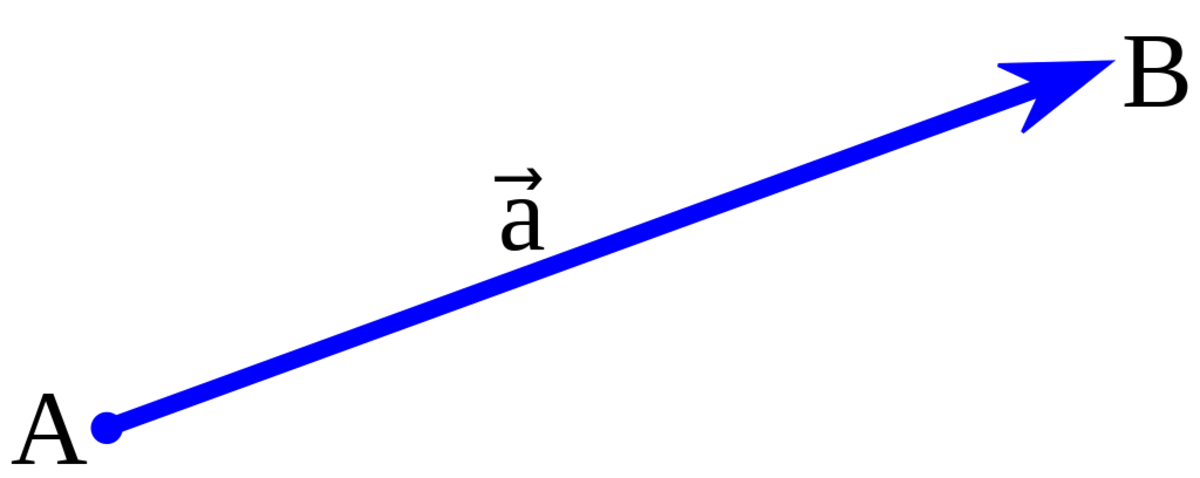Graphical representation of a vector.guyenthephuc , CC BY SA 3.0 via Wikimedia Commons

## Examples of Vectors and Scalars

• Temperature
• Pressure
• Voltage
• Time
• Mass

### Vectors

• Velocity
• Force
• Acceleration
• Magnetic field strength
• Weight

## What Are Vector Diagrams?

In mechanics, we use free-body or force diagrams to describe and sketch the forces in a system. A force is usually represented by an arrow and its direction of action is indicated by the direction of the arrowhead. Rectangles or circles can be used to represent masses.

## How to Add Vectors

As mentioned briefly above, a force or any other vector can be represented graphically by an arrow with a given direction known as a vector. If two or more forces are involved, problems in mechanics can be solved graphically by drawing the vectors as a vector diagram, the head of one vector ending at the tail of the 2nd vector and so on. The vectors are drawn to scale, the length representing the magnitude of a force and the angle being the angle of action of the a. The "Triangle of Forces" or "Parallelogram of Forces" is then a method for visualizing or finding the resultant of forces. The resultant vector from adding force vectors gives the magnitude and direction of two or more forces combined.

### Vector Algebra: The Triangle of Forces

We can add forces graphically using a triangle of forces. When a body is under equilibrium of three forces acting at a point, the forces can be represented by the sides of a triangle taken in order.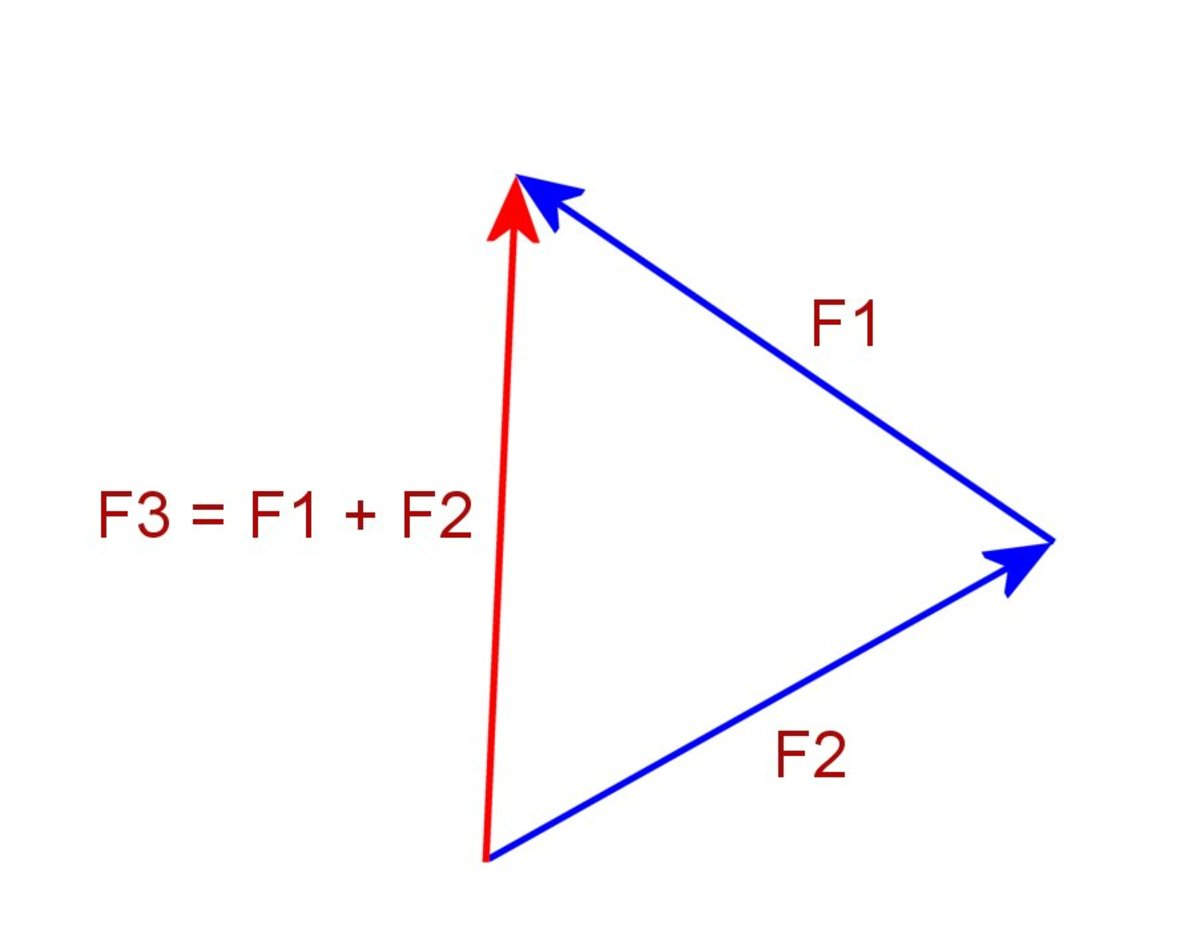A vector diagram showing a triangle of forces. F3 is the sum of the two forces F1 and F2© Eugene Brennan

### Vector Algebra: The Parallelogram of Forces

We can also sum forces using a parallelogram of forces. In the diagram below, one dotted side is drawn parallel to F1 and the other dotted side parallel to F2. A line is drawn joining the start of vectors F1 and F2 to where the two dotted sides intersect. This line represents the sum of the two forces F1 and F2.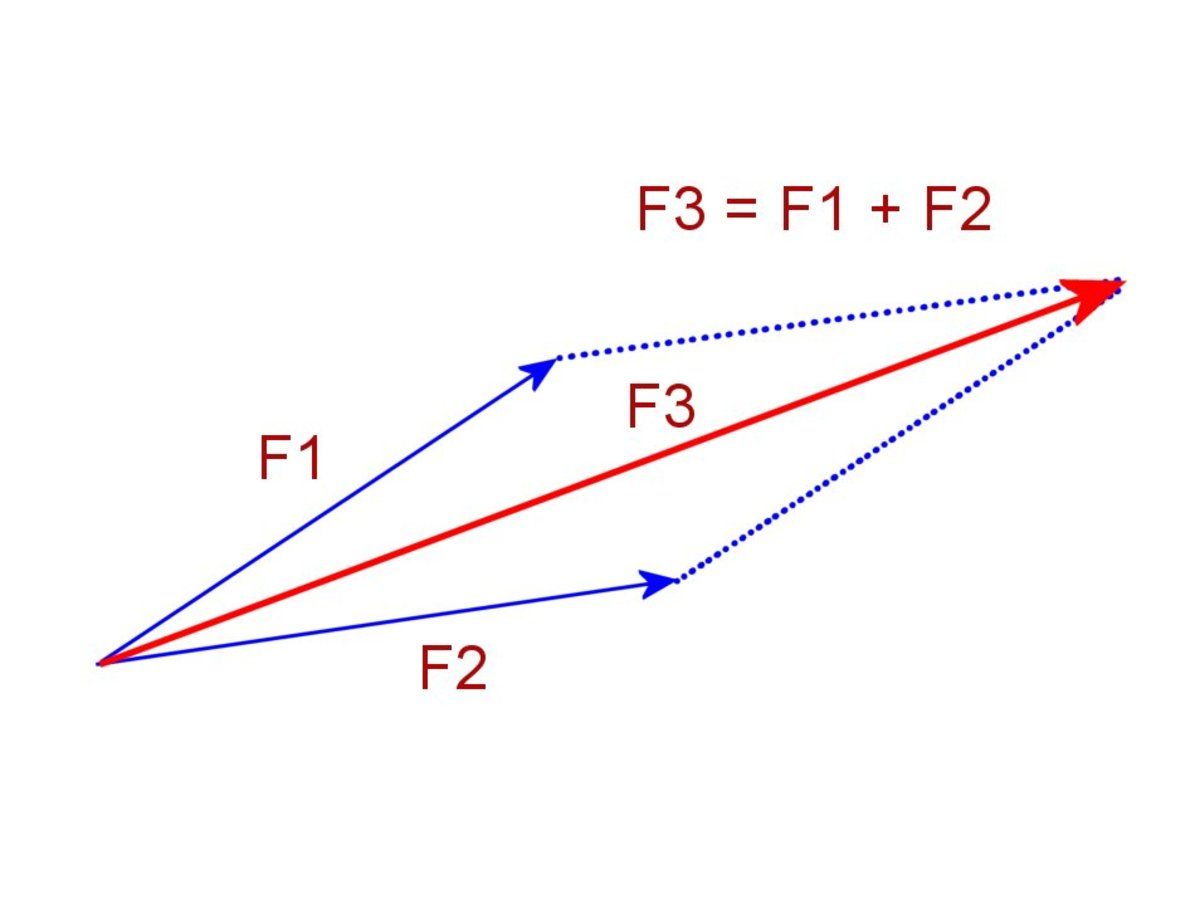Parallelogram of forces. F3 is the sum of the forces F1 and F2© Eugene Brennan

## Resolving Forces

We can also resolve or split up a force into two or more forces. This is like using the parallelogram of forces in reverse. In the diagram below, a mass rests on a slope. Using the parallelogram of forces in reverse, the weight force can be resolved into a force parallel to the slope and perpendicular to the slope. This is useful for these type of problems because it enables the normal reaction to be worked out (the force exerted by the slope on the mass as explained earlier) and also the friction forces involved.

## Using the Parallelogram of Forces to Resolve Weight Into Normal and Tangential Forces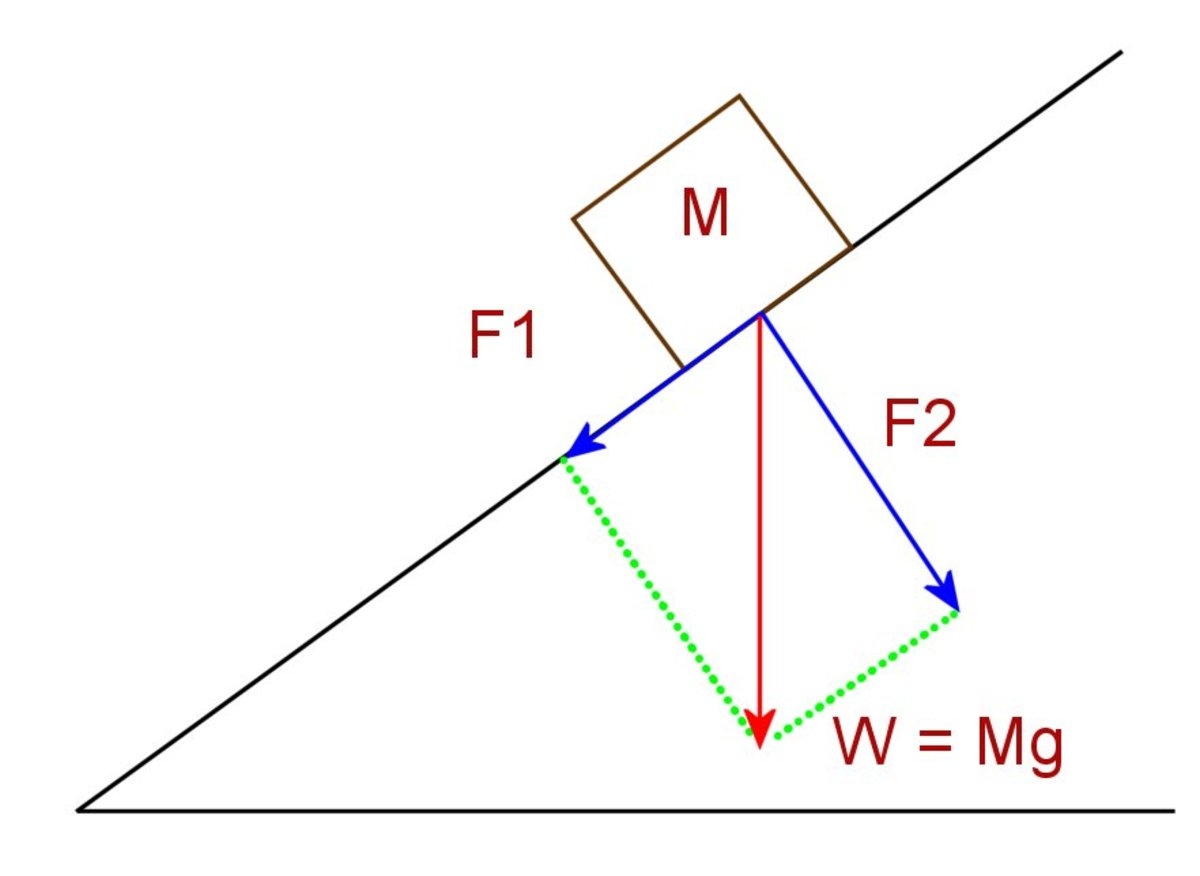Resolving forces for a mass on a slope. The red vector can be replaced by the two blue vectors.© Eugene Brennan

## What Happens When a Force Acts on an Object?

Its velocity (which you'll remember is the same as speed, but in a certain direction) increases. We call an increase in speed, acceleration.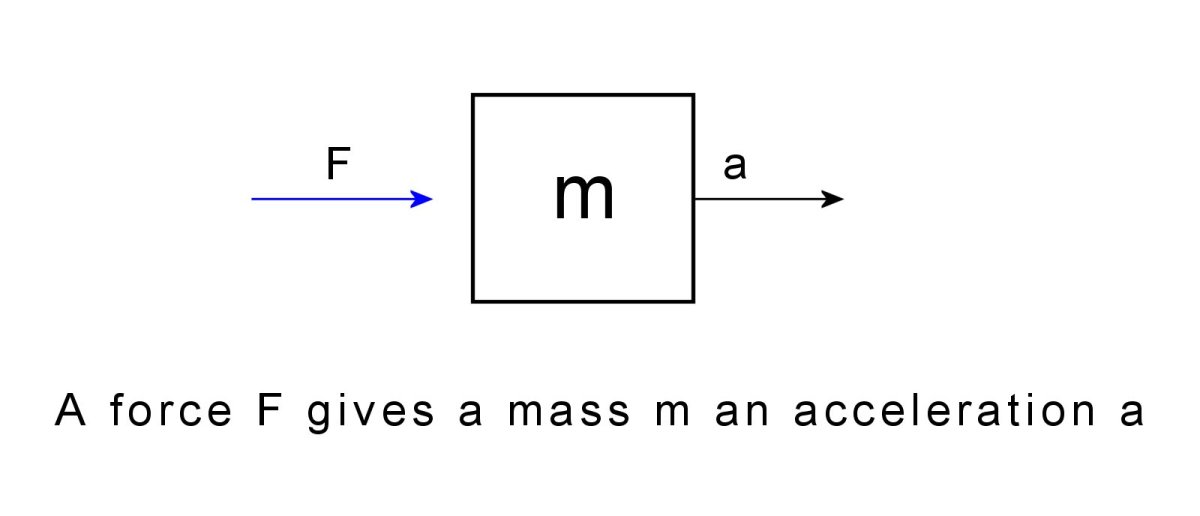A force causes a mass to accelerate© Eugene Brennan

### Newton's Three Laws of Motion

In the second part of this tutorial, we'll cover Newton's Three Laws of Motion for Beginners. This explains in more detail what happens to an object when a force acts on it.

### Mechanics

Applied Mechanics by John Hanah and MJ Hillier is a standard text book for students taking Diploma and Technician courses in engineering. It covers the basic concepts I briefly cover in this article as well as as other topics such as motion in a circle, periodic motion, statics and frameworks, impulse and momentum, stress and strain, bending of beams and fluid dynamics. Worked examples are included in each chapter in addition to set problems with answers provided.

### Mathematics

Engineering Mathematics by K.A. Stroud is an excellent math textbook for both engineering students and anyone with an interest in the subject. The material has been written for part 1 of BSc. Engineering Degrees and Higher National Diploma courses.

A wide range of topics are covered including matrices, vectors, complex numbers, calculus, calculus applications, differential equations, series, probability theory, and statistics. The text is written in the style of a personal tutor, guiding the reader through the content, posing questions, and encouraging them to provide the answer.

This book basically makes learning mathematics fun!

Hannah, J. and Hillerr, M. J., (1971) Applied Mechanics (First metric ed. 1971) Pitman Books Ltd., London, England.

This article is accurate and true to the best of the author’s knowledge. Content is for informational or entertainment purposes only and does not substitute for personal counsel or professional advice in business, financial, legal, or technical matters.

© 2022 Eugene Brennan